# “Space is limited” Trinh Do

In mathematics, four-dimensional space (“4D”) is a geometric space with four dimensions. It is typically meant to mean four-dimensional Euclidean space, generalizing the rules of three-dimensional Euclidean space. It has been studied by mathematicians and philosophers for over two centuries, both for its own interest and for the insights it offered into mathematics and related fields.

Algebraically it is generated by applying the rules of vectors and coordinate geometry to a space with four dimensions. In particular a vector with four elements (a 4-tuple) can be used to represent a position in four-dimensional space. The space is aEuclidean space, so has a metric and norm, and so all directions are treated as the same: the additional dimension is indistinguishable from the other three.

In modern physics, space and time are unified in a four-dimensional Minkowski continuum called spacetime, whose metrictreats the time dimension differently from the three spatial dimensions (see below for the definition of the Minkowski metric/pairing). Spacetime is not a Euclidean space.

## History

Lagrange wrote in his Mécanique analytique (published 1788, based on work done around 1755) that mechanics can be viewed as operating in a four-dimensional space — three of dimensions of space, and one of time. In 1827 Möbius realized that a fourth dimension would allow a three-dimensional form to be rotated onto its mirror-image,and by 1853 Ludwig Schläfli had discovered many polytopes in higher dimensions, although his work was not published until after his death. Higher dimensions were soon put on firm footing by Bernhard Riemann’s 1854 Habilitationsschrift, Über die Hypothesen welche der Geometrie zu Grunde liegen, in which he considered a “point” to be any sequence of coordinates (x1, …, xn). The possibility of geometry in higher dimensions, including four dimensions in particular, was thus established.

An arithmetic of four dimensions called quaternions was defined by William Rowan Hamilton in 1843. This associative algebra was the source of the science of vector analysis in three dimensions as recounted in A History of Vector Analysis.

One of the first major expositors of the fourth dimension was Charles Howard Hinton, starting in 1880 with his essay What is the Fourth Dimension?; published in the Dublin University magazine. He coined the terms tesseractana and kata in his book A New Era of Thought, and introduced a method for visualising the fourth dimension using cubes in the book Fourth Dimension.

In 1908, Hermann Minkowski presented a paper consolidating the role of time as the fourth dimension of spacetime, the basis for Einstein’s theories of special and general relativity. But the geometry of spacetime, being non-Euclidean, is profoundly different from that popularised by Hinton. The study of such Minkowski spaces required new mathematics quite different from that of four-dimensional Euclidean space, and so developed along quite different lines. This separation was less clear in the popular imagination, with works of fiction and philosophy blurring the distinction, so in 1973 H. S. M. Coxeter felt compelled to write:

Little, if anything, is gained by representing the fourth Euclidean dimension as time. In fact, this idea, so attractively developed by H. G. Wells in The Time Machine, has led such authors as John William Dunne (An Experiment with Time) into a serious misconception of the theory of Relativity. Minkowski’s geometry of space-time is not Euclidean, and consequently has no connection with the present investigation.

—H. S. M. Coxeter, Regular Polytopes

## Vectors

Mathematically four-dimensional space is simply a space with four spatial dimensions, that is a space that needs four parameters to specify a point in it. For example, a general point might have position vector a, equal to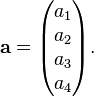$mathbf{a} = begin{pmatrix} a_1 a_2 a_3 a_4 end{pmatrix}.$

This can be written in terms of the four standard basis vectors (e1e2e3e4), given by$mathbf{e}_1 = begin{pmatrix} 1 0 0 0 end{pmatrix}; mathbf{e}_2 = begin{pmatrix} 0 1 0 0 end{pmatrix}; mathbf{e}_3 = begin{pmatrix} 0 0 1 0 end{pmatrix}; mathbf{e}_4 = begin{pmatrix} 0 0 0 1 end{pmatrix},$

so the general vector a is$mathbf{a} = a_1mathbf{e}_1 + a_2mathbf{e}_2 + a_3mathbf{e}_3 + a_4mathbf{e}_4.$

Vectors add, subtract and scale as in three dimensions.

The dot product of Euclidean three-dimensional space generalizes to four dimensions as$mathbf{a} cdot mathbf{b} = a_1 b_1 + a_2 b_2 + a_3 b_3 + a_4 b_4.$

It can be used to calculate the norm or length of a vector,$left| mathbf{a} right| = sqrt{mathbf{a} cdot mathbf{a} } = sqrt{{a_1}^2 + {a_2}^2 + {a_3}^2 + {a_4}^2},$

and calculate or define the angle between two vectors as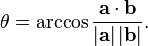$theta = arccos{frac{mathbf{a} cdot mathbf{b}}{left|mathbf{a}right| left|mathbf{b}right|}}.$

Minkowski spacetime is four-dimensional space with geometry defined by a nondegenerate pairing different from the dot product: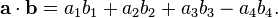$mathbf{a} cdot mathbf{b} = a_1 b_1 + a_2 b_2 + a_3 b_3 - a_4 b_4.$

As an example, the distance squared between the points (0,0,0,0) and (1,1,1,0) is 3 in both the Euclidean and Minkowskian 4-spaces, while the distance squared between (0,0,0,0) and (1,1,1,1) is 4 in Euclidean space and 2 in Minkowski space; increasing$b_4$ actually decreases the metric distance. This leads to many of the well known apparent “paradoxes” of relativity.

The cross product is not defined in four dimensions. Instead the exterior product is used for some applications, and is defined as follows:begin{align} mathbf{a} wedge mathbf{b} = (a_1b_2 - a_2b_1)mathbf{e}_{12} + (a_1b_3 - a_3b_1)mathbf{e}_{13} + (a_1b_4 - a_4b_1)mathbf{e}_{14} + (a_2b_3 - a_3b_2)mathbf{e}_{23} + (a_2b_4 - a_4b_2)mathbf{e}_{24} + (a_3b_4 - a_4b_3)mathbf{e}_{34}. end{align}

This is bivector valued, with bivectors in four dimensions forming a six-dimensional linear space with basis (e12e13e14e23e24e34). They can be used to generate rotations in four dimensions.

## Orthogonality and vocabulary

In the familiar 3-dimensional space that we live in there are three coordinate axes — usually labeled xy, and z — with each axis orthogonal (i.e. perpendicular) to the other two. The six cardinal directions in this space can be called updowneastwestnorth, and south. Positions along these axes can be called altitudelongitude, and latitude. Lengths measured along these axes can be called heightwidth, and depth.

Comparatively, 4-dimensional space has an extra coordinate axis, orthogonal to the other three, which is usually labeled w. To describe the two additional cardinal directions,Charles Howard Hinton coined the terms ana and kata, from the Greek words meaning “up toward” and “down from”, respectively. A length measured along the w axis can be called spissitude, as coined by Henry More.

## Geometry

The geometry of 4-dimensional space is much more complex than that of 3-dimensional space, due to the extra degree of freedom.

Just as in 3 dimensions there are polyhedra made of two dimensional polygons, in 4 dimensions there are polychora (4-polytopes) made of polyhedra. In 3 dimensions there are 5 regular polyhedra known as the Platonic solids. In 4 dimensions there are 6 convex regular polychora, the analogues of the Platonic solids. Relaxing the conditions for regularity generates a further 58 convex uniform polychora, analogous to the 13 semi-regular Archimedean solids in three dimensions.

Regular polytopes in four dimensions
(Displayed as orthogonal projections in each Coxeter plane of symmetry)
A4 BC4 F4 H4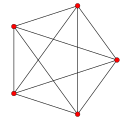5-cell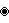tesseract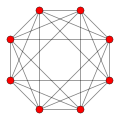16-cell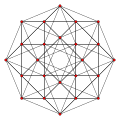24-cell120-cell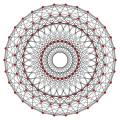600-cellIn 3 dimensions, a circle may be extruded to form a cylinder. In 4 dimensions, there are several different cylinder-like objects. A sphere may be extruded to obtain a spherical cylinder (a cylinder with spherical “caps”), and a cylinder may be extruded to obtain a cylindrical prism. The Cartesian product of two circles may be taken to obtain aduocylinder. All three can “roll” in 4-dimensional space, each with its own properties.

In 3 dimensions, curves can form knots but surfaces cannot (unless they are self-intersecting). In 4 dimensions, however, knots made using curves can be trivially untied by displacing them in the fourth direction, but 2-dimensional surfaces can form non-trivial, non-self-intersecting knots in 4-dimensional space. Because these surfaces are 2-dimensional, they can form much more complex knots than strings in 3-dimensional space can. The Klein bottle is an example of such a knotted surface .[citation needed]Another such surface is the real projective plane.[citation needed]

### Hypersphere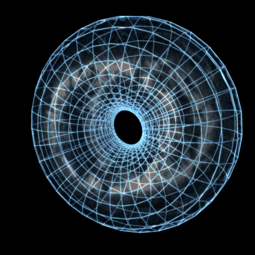Stereographic projection of a Clifford torus: the set of points (cos(a), sin(a), cos(b), sin(b)), which is a subset of the 3-sphere.

The set of points in Euclidean 4-space having the same distance R from a fixed point P0 forms a hypersurface known as a 3-sphere. The hyper-volume of the enclosed space is: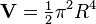$mathbf V = begin{matrix} frac{1}{2} end{matrix} pi^2 R^4$

This is part of the Friedmann–Lemaître–Robertson–Walker metric in General relativity where R is substituted by function R(t)with t meaning the cosmological age of the universe. Growing or shrinking R with time means expanding or collapsing universe, depending on the mass density inside.

## Cognition

Research using virtual reality finds that humans in spite of living in a three-dimensional world can without special practice make spatial judgments based on the length of, and angle between, line segments embedded in four-dimensional space. The researchers noted that “the participants in our study had minimal practice in these tasks, and it remains an open question whether it is possible to obtain more sustainable, definitive, and richer 4D representations with increased perceptual experience in 4D virtual environments.” In another study, the ability of humans to orient themselves in 2D, 3D and 4D mazes has been tested. Each maze consisted of four path segments of random length and connected with orthogonal random bends, but without branches or loops (i.e. actually labyrinths). The graphical interface was based on John McIntosh’s free 4D Maze game. The participating persons had to navigate through the path and finally estimate the linear direction back to the starting point. The researchers found that some of the participants were able to mentally integrate their path after some practice in 4D (the lower-dimensional cases were for comparison and for the participants to learn the method).

## Dimensional analogyA net of a tesseract

To understand the nature of four-dimensional space, a device called dimensional analogy is commonly employed. Dimensional analogy is the study of how (n − 1) dimensions relate to n dimensions, and then inferring how n dimensions would relate to (n + 1) dimensions.

Dimensional analogy was used by Edwin Abbott Abbott in the book Flatland, which narrates a story about a square that lives in a two-dimensional world, like the surface of a piece of paper. From the perspective of this square, a three-dimensional being has seemingly god-like powers, such as ability to remove objects from a safe without breaking it open (by moving them across the third dimension), to see everything that from the two-dimensional perspective is enclosed behind walls, and to remain completely invisible by standing a few inches away in the third dimension.

By applying dimensional analogy, one can infer that a four-dimensional being would be capable of similar feats from our three-dimensional perspective. Rudy Rucker illustrates this in his novel Spaceland, in which the protagonist encounters four-dimensional beings who demonstrate such powers.

## Cross-sections

As a three-dimensional object passes through a two-dimensional plane, a two-dimensional being would only see a cross-section of the three-dimensional object. For example, if a balloon passed through a sheet of paper, a being on the paper would see a circle gradually grow larger, then smaller again. Similarly, if a four-dimensional object passed through three-dimensions, we would see a three-dimensional cross-section of the four-dimensional object–for example, a sphere.

### Projections

A useful application of dimensional analogy in visualizing the fourth dimension is in projection. A projection is a way for representing an n-dimensional object in n − 1 dimensions. For instance, computer screens are two-dimensional, and all the photographs of three-dimensional people, places and things are represented in two dimensions by projecting the objects onto a flat surface. When this is done, depth is removed and replaced with indirect information. The retina of the eye is also a two-dimensional arrayof receptors but the brain is able to perceive the nature of three-dimensional objects by inference from indirect information (such as shading, foreshortening, binocular vision, etc.). Artists often use perspective to give an illusion of three-dimensional depth to two-dimensional pictures.

Similarly, objects in the fourth dimension can be mathematically projected to the familiar 3 dimensions, where they can be more conveniently examined. In this case, the ‘retina’ of the four-dimensional eye is a three-dimensional array of receptors. A hypothetical being with such an eye would perceive the nature of four-dimensional objects by inferring four-dimensional depth from indirect information in the three-dimensional images in its retina.

The perspective projection of three-dimensional objects into the retina of the eye introduces artifacts such as foreshortening, which the brain interprets as depth in the third dimension. In the same way, perspective projection from four dimensions produces similar foreshortening effects. By applying dimensional analogy, one may infer four-dimensional “depth” from these effects.

As an illustration of this principle, the following sequence of images compares various views of the 3-dimensional cube with analogous projections of the 4-dimensional tesseract into three-dimensional space.

Cube Tesseract DescriptionThe image on the left is a cube viewed face-on. The analogous viewpoint of the tesseract in 4 dimensions is the cell-first perspective projection, shown on the right. One may draw an analogy between the two: just as the cube projects to a square, the tesseract projects to a cube.

Note that the other 5 faces of the cube are not seen here. They are obscured by the visible face. Similarly, the other 7 cells of the tesseract are not seen here because they are obscured by the visible cell.The image on the left shows the same cube viewed edge-on. The analogous viewpoint of a tesseract is the face-first perspective projection, shown on the right. Just as the edge-first projection of the cube consists of two trapezoids, the face-first projection of the tesseract consists of two frustums.The nearest edge of the cube in this viewpoint is the one lying between the red and green faces. Likewise, the nearest face of the tesseract is the one lying between the red and green cells.On the left is the cube viewed corner-first. This is analogous to the edge-first perspective projection of the tesseract, shown on the right. Just as the cube’s vertex-first projection consists of 3 deltoids surrounding a vertex, the tesseract’s edge-first projection consists of 3 hexahedral volumes surrounding an edge. Just as the nearest vertex of the cube is the one where the three faces meet, so the nearest edge of the tesseract is the one in the center of the projection volume, where the three cells meet.A different analogy may be drawn between the edge-first projection of the tesseract and the edge-first projection of the cube. The cube’s edge-first projection has two trapezoids surrounding an edge, while the tesseract has three hexahedral volumes surrounding an edge.On the left is the cube viewed corner-first. The vertex-first perspective projection of the tesseract is shown on the right. The cube’s vertex-first projection has three tetragons surrounding a vertex, but the tesseract’s vertex-first projection has four hexahedral volumes surrounding a vertex. Just as the nearest corner of the cube is the one lying at the center of the image, so the nearest vertex of the tesseract lies not on boundary of the projected volume, but at its centerinside, where all four cells meet.

Note that only three faces of the cube’s 6 faces can be seen here, because the other 3 lie behind these three faces, on the opposite side of the cube. Similarly, only 4 of the tesseract’s 8 cells can be seen here; the remaining 4 lie behindthese 4 in the fourth direction, on the far side of the tesseract.

If the wireframe of a cube is lit from above, the resulting shadow is a square within a square with the corresponding corners connected. Similarly, if the wireframe of a tesseract were lit from “above” (in the fourth direction), its shadow would be that of a three-dimensional cube within another three-dimensional cube. (Note that, technically, the visual representation shown here is actually a two-dimensional shadow of the three-dimensional shadow of the four-dimensional wireframe figure.

### Bounding volumes

Dimensional analogy also helps in inferring basic properties of objects in higher dimensions. For example, two-dimensional objects are bounded by one-dimensional boundaries: a square is bounded by four edges. Three-dimensional objects are bounded by two-dimensional surfaces: a cube is bounded by 6 square faces. By applying dimensional analogy, one may infer that a four-dimensional cube, known as a tesseract, is bounded by three-dimensional volumes. And indeed, this is the case: mathematics shows that the tesseract is bounded by 8 cubes. Knowing this is key to understanding how to interpret a three-dimensional projection of the tesseract. The boundaries of the tesseract project to volumes in the image, not merely two-dimensional surfaces.

### Visual scope

Being three-dimensional, we are only able to see the world with our eyes in two dimensions. A four-dimensional being would be able to see the world in three dimensions. For example, it would be able to see all six sides of an opaque box simultaneously, and in fact, what is inside the box at the same time, just as we can see the interior of a square on a piece of paper. It would be able to see all points in 3-dimensional space simultaneously, including the inner structure of solid objects and things obscured from our three-dimensional viewpoint. Our brains receive images in the second dimension and use reasoning to help us “picture” three-dimensional objects. Just as a four-dimensional creature would probably receive multiple three-dimensional pictures.

### Limitations

Reasoning by analogy from familiar lower dimensions can be an excellent intuitive guide, but care must be exercised not to accept results that are not more rigorously tested. For example, consider the formulas for the circumference of a circle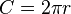$C = 2pi r$ and the surface area of a sphere:$A = 4pi r^2$. One might be tempted to suppose that the surface volume of a hypersphere is$V=6pi r^3$, or perhaps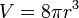$V=8pi r^3$, but either of these would be wrong. The correct formula is$V = 2pi^2 r^3$.